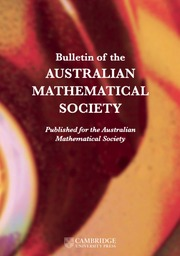Home
Hostname: page-component-768dbb666b-7lw58 Total loading time: 0.685 Render date: 2023-02-03T21:34:40.904Z Has data issue: true Feature Flags: { "useRatesEcommerce": false } hasContentIssue trueBulletin of the Australian Mathematical Society

## Abstract

Given$E \subseteq \mathbb {F}_q^d \times \mathbb {F}_q^d$ , with the finite field$\mathbb {F}_q$ of order q and the integer$d\,\ge \, 2$ , we define the two-parameter distance set$\Delta _{d, d}(E)=\{(\|x-y\|, \|z-t\|) : (x, z), (y, t) \in E \}$ . Birklbauer and Iosevich [‘A two-parameter finite field Erdős–Falconer distance problem’, Bull. Hellenic Math. Soc.61 (2017), 21–30] proved that if$|E| \gg q^{{(3d+1)}/{2}}$ , then$|\Delta _{d, d}(E)| = q^2$ . For$d=2$ , they showed that if$|E| \gg q^{{10}/{3}}$ , then$|\Delta _{2, 2}(E)| \gg q^2$ . In this paper, we give extensions and improvements of these results. Given the diagonal polynomial$P(x)=\sum _{i=1}^da_ix_i^s\in \mathbb F_q[x_1,\ldots , x_d]$ , the distance induced by P over$\mathbb {F}_q^d$ is$\|x-y\|_s:=P(x-y)$ , with the corresponding distance set$\Delta ^s_{d, d}(E)=\{(\|x-y\|_s, \|z-t\|_s) : (x, z), (y, t) \in E \}$ . We show that if$|E| \gg q^{{(3d+1)}/{2}}$ , then$|\Delta _{d, d}^s(E)| \gg q^2$ . For$d=2$ and the Euclidean distance, we improve the former result over prime fields by showing that$|\Delta _{2,2}(E)| \gg p^2$ for$|E| \gg p^{{13}/{4}}$ .

## MSC classification

Type
Research Article
© The Author(s), 2022. Published by Cambridge University Press on behalf of Australian Mathematical Publishing Association Inc.

## Access options

Get access to the full version of this content by using one of the access options below. (Log in options will check for institutional or personal access. Content may require purchase if you do not have access.)

## Footnotes

The second-listed author was supported by Swiss National Science Foundation grant P2ELP2-178313.

## References

Birklbauer, P. and Iosevich, A., ‘A two-parameter finite field Erdős–Falconer distance problem’, Bull. Hellenic Math. Soc. 61 (2017), 2130.Google Scholar
Bourgain, J., Katz, N. and Tao, T., ‘A sum-product estimate in finite fields, and applications’, Geom. Funct. Anal. 14 (2004), 2757.CrossRefGoogle Scholar
Chapman, J., Erdogan, M., Hart, D., Iosevich, A. and Koh, D., ‘Pinned distance sets,$k$ -simplices, Wolff’s exponent in finite fields and sum-product estimates’, Math. Z. 271 (2012), 6393.CrossRefGoogle Scholar
Du, X., Ou, Y. and Zhang, R., ‘On the multiparameter Falconer distance problem’, Trans. Amer. Math. Soc. 375 (2022), 49795010.CrossRefGoogle Scholar
Hambrook, K., Iosevich, A. and Rice, A., ‘Group actions and a multi-parameter Falconer distance problem’, Preprint, 2017, arXiv:1705.03871.Google Scholar
Hart, D., Iosevich, A., Koh, D. and Rudnev, M., ‘Averages over hyperplanes, sum-product theory in vector spaces over finite fields and the Erdös–Falconer distance conjecture’, Trans. Amer. Math. Soc. 363 (2011), 32553275.CrossRefGoogle Scholar
Hieu, D. and Pham, T., ‘Distinct distances on regular varieties over finite fields’, J. Number Theory 173 (2017), 602613.CrossRefGoogle Scholar
Iosevich, A., Janczak, M. and Passant, J., ‘A multi-parameter variant of the Erdős distance problem’, Preprint, 2017, arXiv:1712.04060.Google Scholar
Iosevich, A. and Rudnev, M., ‘Erdős distance problem in vector spaces over finite fields’, Trans. Amer. Math. Soc. 359 (2007), 61276142.CrossRefGoogle Scholar
Koh, D., Pham, T. and Vinh, L., ‘Extension theorems and a connection to the Erdős–Falconer distance problem over finite fields’, J. Funct. Anal. 281 (2021), 154.CrossRefGoogle Scholar
Koh, D. and Shen, C.-Y., ‘The generalized Erdős–Falconer distance problems in vector spaces over finite fields’, J. Number Theory 132 (2012), 24552473.CrossRefGoogle Scholar
Murphy, B., Petridis, G., Pham, T., Rudnev, M. and Stevens, S., ‘On the pinned distances problem in positive characteristic’, J. Lond. Math. Soc. (2) 105 (2022), 469499.CrossRefGoogle Scholar
Pham, T. and Suk, A., ‘Structures of distance sets over prime fields’, Proc. Amer. Math. Soc. 148 (2020), 32093215.CrossRefGoogle Scholar
Pham, T. and Vinh, L., ‘Distribution of distances in vector spaces over prime fields’, Pacific J. Math. 309 (2020), 437451.CrossRefGoogle Scholar
Vinh, L., ‘On the generalized Erdős–Falconer distance problems over finite fields’, J. Number Theory 133 (2013), 29392947.CrossRefGoogle Scholar

# Save article to Kindle

Note you can select to save to either the @free.kindle.com or @kindle.com variations. ‘@free.kindle.com’ emails are free but can only be saved to your device when it is connected to wi-fi. ‘@kindle.com’ emails can be delivered even when you are not connected to wi-fi, but note that service fees apply.

Find out more about the Kindle Personal Document Service.

ON THE TWO-PARAMETER ERDŐS–FALCONER DISTANCE PROBLEM IN FINITE FIELDS
Available formats
×

# Save article to Dropbox

To save this article to your Dropbox account, please select one or more formats and confirm that you agree to abide by our usage policies. If this is the first time you used this feature, you will be asked to authorise Cambridge Core to connect with your Dropbox account. Find out more about saving content to Dropbox.

ON THE TWO-PARAMETER ERDŐS–FALCONER DISTANCE PROBLEM IN FINITE FIELDS
Available formats
×

# Save article to Google Drive

To save this article to your Google Drive account, please select one or more formats and confirm that you agree to abide by our usage policies. If this is the first time you used this feature, you will be asked to authorise Cambridge Core to connect with your Google Drive account. Find out more about saving content to Google Drive.

ON THE TWO-PARAMETER ERDŐS–FALCONER DISTANCE PROBLEM IN FINITE FIELDS
Available formats
×
×